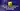Do you want to become a better fullstack software engineer?
<->Discover how to select a random element from an array in JavaScript.

Let's imagine that you created a giveaway application, and today is the raffle. Unfortunately, you have a list of 10 participants, but you don't know how to select randomly one of them as a winner.

Don't worry! In a few minutes, you will be able to get a random element from an array in JavaScript!

## How to Select a Random Element from an Array using the Mathematical functions

Here is the one line instruction to get a a random element from your array: `YOUR_ARRAY[Math.floor(Math.random() * YOUR_ARRAY.length)]`.

Let's break this instruction and understand what it does:

• `YOUR_ARRAY` is your array variable (in that case, the 10 participants email addresses)
• `YOUR_ARRAY.length` is an array property that returns the size of your array
• `Math.random()` is a function that returns a pseudo-random number in the range 0 to less than 1 (inclusive of 0, but not 1)
• `Math.floor()` is a function that returns the largest integer less than or equal to a given number

Note: As Mozilla mentions, `Math.random()` does not provide cryptographically secure random numbers. It's not recommended to use them for anything related to security. Use the Web Crypto API instead, and more precisely, the window.crypto.getRandomValues() method.

Now you know each instruction, let me show you a step-by-step example:

``````const participants = [
'[email protected]',
'[email protected]',
'[email protected]',
'[email protected]',
'[email protected]wecode.io',
'[email protected]',
'[email protected]',
'[email protected]',
'[email protected]',
'[email protected]',
]

console.log(participants.length)
// Output: 10

console.log(Math.random())
// Output: random number between 0 or 1 (ex: 0.623242121481016)

console.log(Math.random() * participants.length)
// Output: random number between 0 or 1 multiplied by 10 (ex: 0.623242121481016 * 10 = 1.6905986987355703)

console.log(Math.floor(Math.random() * participants.length))
// Output: it takes the larger integer less than or equal to a given number (ex: Math.floor(1.6905986987355703) = 1)``````

Note: If you try the code above, the result will always be different because of the `Math.random()` function.

Here we are! It's time to select the giveaway winner! To do so, we will use what we learned in this article and use it with your use case:

``````const participants = [
'[email protected]',
'[email protected]',
'[email protected]',
'[email protected]',
'[email protected]',
'[email protected]',
'[email protected]',
'[email protected]e.io',
'[email protected]',
'[email protected]',
] // 10 participants

const winner = participants[Math.floor(Math.random() * participants.length)]

console.log(winner)
// Output is random (launch this code to see who is the winner :D)``````

So! Who won the jackpot? 😉

➡️ I regularly deliver content about web development, personal growth as a developer, and my journey as an aspiring digital nomad and remote software engineer. If you don't want to miss them, I invite you to follow me on Twitter. 🚀

Learn to code with simple and concrete examples.